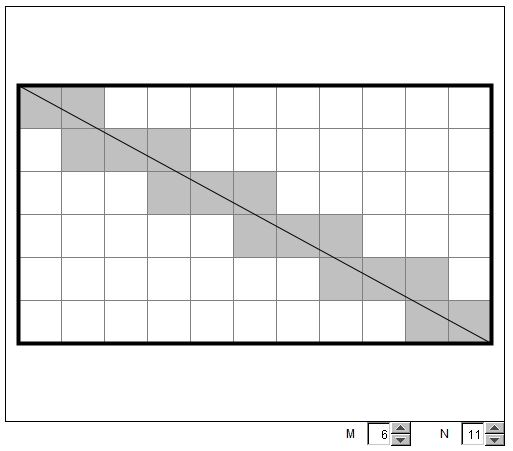# A Line in a Square Grid

On squared paper, draw a rectangle and one of its diagonals. How many grid squares are crossed by the diagonal?

### If you are reading this, your browser is not set to run Java applets. Try IE11 or Safari and declare the site https:///www.cut-the-knot.org as trusted in the Java setup.What if applet does not run?

Solution### Solution

The best way to approach this problem seems to start with a few small rectangles 2×3, 2×5, 3×5, etc. Tabulate the results and next try to generalize. There are going to be exceptional cases, like 2×4, 3×6, 4×6. These would need a special treatment and further generalization.What makes some cases exceptional? The fact that the diagonal passes through grid nodes. When this happens the number of crossed squares appears to be less than for the grids with nearby dimensions. Let's disregard such cases for a moment and focus on the grids where the diagonal does not pass through any of the grid points.

How can we count the crossed squares in that (simple) case? The upper left square is always crossed. This is 1. Next, on its way to the next square the diagonal crosses one of the (internal) grid lines. In fact, this happens every time we move from one crossed square to the next. When we move from one crossed square to the next we step over their common side which is necessarily crossed by the diagonal. It follows that

The number of crossed squares is 1 more than the total number of internal grid lines - both vertical and horizontal - crossed by the diagonal.

But on its way from the upper left corner of the rectangle to the lower right corner, the diagonal crosses all internal vertical as well as horizontal lines. Naturally, each line is crossed only once. How many of these lines are there? For the M×N rectangle, there are (M-1) horizontal and (N-1) vertical lines. Thus the total number of internal lines crossed by the diagonal is L = (M-1) + (N-1) = M + N - 2. As we have already observed, the number S of the crossed squares is 1 more than that:

(1)

S = L + 1 = M + N - 2 + 1 = M + N - 1.

It's a good idea now to check this formula by counting for a few additional cases of (relatively) small M and N. In preparation for the next step, pay attention to those M and N, for which the formula fails, i.e., M and N for which the diagonal passes through (internal) grid points. It's hard to miss the point that this happens when M and N have non-trivial common divisors, i.e., when gcd(M, N) > 1.

It is easy to generalize again. How many internal grid points lie on the diagonal? gcd(M, N) - 1. Had the diagonal been moved just a little bit to avoid those grid points, the formula S = M + N - 1 would have been true. But such a shift would have added 1 count at every grid point on the diagonal. Taking this into account we arrive at another formula:

S = M + N - 1 - (gcd(M, N) - 1) = M + N - gcd(M, N).

In other words, the general formula for the number S of crossed squares is

(2)

S = M + N - gcd(M, N).

Since gcd(M, N) = 1 when M and N have no common factors, meaning geometrically that no grid points lie on the diagonal, (2) is a clear generalization of (1).

(Further generalization would be to consider the configuration where the grid lines are not distributed evenly. In this case, the formula (1) is still true as long as no grid points lie on the diagonal, whereas (2) loses its significance. From this point of view, (1) which is applicable in a more broad context, is more general than (2).)

### References

1. J. Mason, L. Burton, K. Stacey, Thinking Mathematically, Addison-Wesley, 1985• Factoring with the Factor Tree
• GCD and LCM via Factor Tree
• GCD and LCM by Plain Factorization
• Common Multiples and the Least Common Multiple
• GCD(M, N) x LCM(M, N) = M x N
• Divisibility Criteria
• Euclid's Algorithm
• Algorithm for Computing the LCM
• Factors And Multiples
• Two Properties of Greatest Common Divisor
• Properties of GCD and LCM
• Number of Factors of an Integer
• Extension of Euclid's Game
•